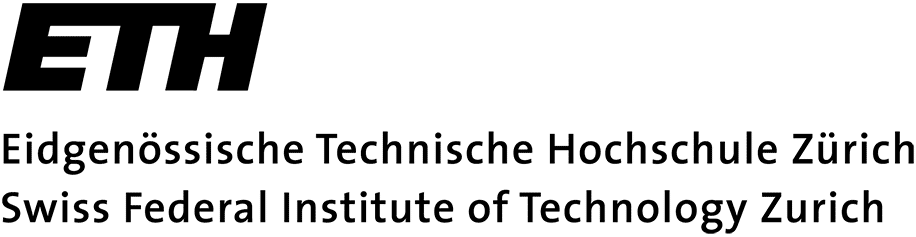## Numerical Methods for Partial Differential Equations

Check in the VVZ for a current information.

Ferienpräsenz: we offer consultation hours during the semester break where you can ask question about the lecture material. For the dates see  this document (in German). It also lists the dates for the Prüfungseinsicht.

Lecturer: Siddhartha Mishra

Assistants: Jonas Sukys, Laura Scarabosio, Simon Etter

Lecture hours:

• Monday, 15-17, HG G 5
• Tuesday, 15-17, HG G 5

Exercise classes:

• INFK: Thursday, 13-15, HG E 41 (Jonas Sukys, Laura Scarabosio)
• INFK: Friday, 08-10, HG E 33.3 (Jonas Sukys, Laura Scarabosio)
• CSE: Friday, 08-10, HG G 26.5 (Simon Etter)

For INFK students, please choose an exercise class here.

Consultation hours:

Consultation hours will be announced in the first exercise class.

### Assignments

There will be assignment sheets every two weeks, handed out every Monday (first time on February 24th).

You can hand in your solutions either during the exercise class or in the labeled box at HG G 53.X.
Correction of homework problems will be done on request.
Remark: in general, the codes will not be corrected, but you can approach us with a specific question.

Testat requirements: NONE.

Bonus points: from the second assignment on, each assignment sheet will contain a problem marked as Core problem. The core problems will be corrected and full mark for them will give a 20% bonus of the total points in the final exam.

For submitting your code, please use the online submission interface and choose the course n....

Assignments, lecture notes and other handouts

### Examination

Info will be given during the first lecture.

At least one of the problems presented in the assignment sheets will be an exam problem.

### Aims of the course

Introduce students of Applied Mathematics, Computational Science and Engineering and Computer Science to the most widely used numerical solution methods for ordinary and partial differential equations, their mathematical properties, and their computer implementation.

Students should be able to:

• implement advanced numerical methods for the solution of partial differential equations efficiently.
• modify and adapt numerical algorithms guided by awareness of their mathematical foundations.
• select and assess numerical methods in light of the predictions of theory.
• identify features of a PDE (= partial differential equation) based model
that are relevant for the selection and performance of a numerical
algorithm.
• understand research publications on theoretical and practical aspects of numerical methods for partial differential equations.

Programming Language: implementations can be done in MATLAB, Python, C or C++.

### Content of the course

• 2-point boundary value problems
• Elliptic boundary value problems
• Parabolic boundary value problems
• Hyperbolic problems

See a more comprehensive list on the VVZ.

Here is an introduction to Python: Python introduction.

### Literature

This list should be interpreted as supplementary reading beyond the lecture notes, and is neither important nor required for following the course.

• W. Bangerth and R. Rannacher, Adaptive Finite Element Methods for Differential Equations, Lectures in Mathematics, ETH Zürich, Birkhäuser, 2003.
• D. Braess, Finite Elements, 2nd Edition, Cambridge University Press, 2001.
• S. Brenner and R. Scott, Mathematical theory of finite element methods, Texts in Applied Mathematics. Springer–Verlag, New York, 1994.
• A. Ern and J.-L. Guermond Theory and Practice of Finite Elements, volume 159 of Applied Mathematical Sciences. Springer, New York, 2004.
• Ch. Großmann and H.-G. Roos, Numerik partieller Differentialgleichungen, Teubner Studienbücher Mathematik. Teubner, Stuttgart, 1992.
• W. Hackbusch, Elliptic Differential Equations. Theory and Numerical Treatment, volume 18 of Springer Series in Computational Mathematics. Springer, Berlin, 1992.
• T. Hughes, The Finite Element Method, Dover Publications, 2000.
• C. Johnson, Numerical Solution of Partial Differential Equations by the Finite Element Method, Cambridge University Press, 1987.
• P. Knabner and L. Angermann, Numerical Methods for Elliptic and Parabolic Partial Differential Equations, volume 44 of Texts in Applied Mathematics. Springer, Heidelberg, 2003.
• S. Larsson and V. Thomée, Partial Differential Equations with Numerical Methods, volume 45 of Texts in Applied Mathematics. Springer, Heidelberg, 2003.
• R. LeVeque, Finite Volume Methods for Hyperbolic Problems, Cambridge University Press, 2002.
• A. Quarteroni, R. Sacco und F. Salieri, Numerische Mathematik, Springer, 2002.

### Further literature

These are beyond the scope of this course, and are listed here for the particularly interested.

• C. Canuto, M.Y. Hussaini, A. Quarteroni und T.A. Zang, Spectral Methods, Springer, 2006.
• M. Feistauer, J. Felcman, and I. Straskraba, Mathematical and Computational Methods for Compressible Flow, Oxford: Clarendon Press, 2003.
• A. Tveito und R. Winther, Einführung in Partielle Differentialgleichungen, Springer, 2002.

Wichtiger Hinweis:
Diese Website wird in älteren Versionen von Netscape ohne graphische Elemente dargestellt. Die Funktionalität der Website ist aber trotzdem gewährleistet. Wenn Sie diese Website regelmässig benutzen, empfehlen wir Ihnen, auf Ihrem Computer einen aktuellen Browser zu installieren. Weitere Informationen finden Sie auf
folgender Seite.

Important Note:
The content in this site is accessible to any browser or Internet device, however, some graphics will display correctly only in the newer versions of Netscape. To get the most out of our site we suggest you upgrade to a newer browser.